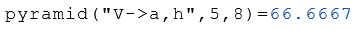# Class Pyramid

Calculation of the properties of pyramids

## Description

The class Pyramid calculates properties of regular pyramids.

## Properties

The following properties can be queried as a result or passed as an argument.

#### Description

Base area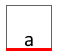a Side length of the base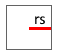rs Radius from the center to a straight line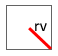rv Radius from the center to a corner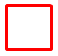P Perimeter of the base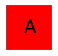A Area of the base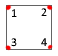n Number of corners. The specification is optional. The default is 4.
Dimensions of the pyramid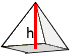h Height of the pyramid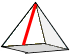L Height of a side
Areas and volumes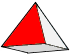Sl Surface of a side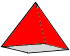Sa Surface without base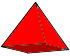S Total surface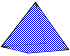V Volume

## Syntax

Object = Pyramid ("arg names", arguments)

Value = Pyramid ("get -> arg names", arguments)

## Example

Calculation of the properties of the side length $$a$$ and the height $$h$$.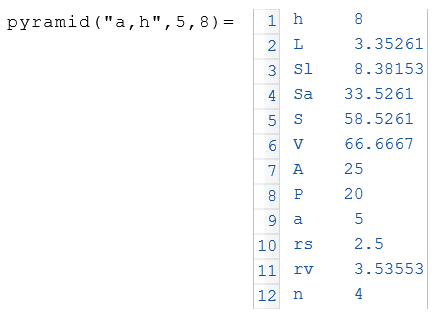The following example displays the volume $$V$$ from the object $$a$$.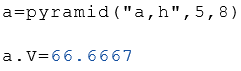Calculation of the volume $$V$$.